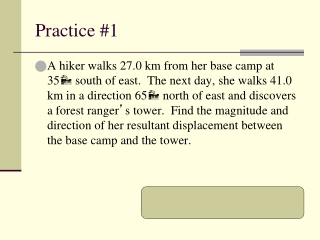DownloadDownload PresentationPractice #1

# Practice #1

Download Presentation## Practice #1

- - - - - - - - - - - - - - - - - - - - - - - - - - - E N D - - - - - - - - - - - - - - - - - - - - - - - - - - -
##### Presentation Transcript

1. Practice #1 • A hiker walks 27.0 km from her base camp at 35 south of east. The next day, she walks 41.0 km in a direction 65 north of east and discovers a forest ranger’s tower. Find the magnitude and direction of her resultant displacement between the base camp and the tower. Answer ~ 45 km at 29 degrees N of E

2. Non-perpendicular vectors • Because vectors do not always form right triangles, you cannot automatically apply the Pythagorean theorem and tangent function to the original vectors Vector 2 Resultant vector No right triangle! Vector 1

3. Non-perpendicular vectors • Step #1 You must resolve vector #1 into x & y components Vector 1 DR1 Δy1 θ1 Δx1

4. Non-perpendicular vectors • Step #2 You must resolve vector #2 into x & y components DR2 Δy2 Vector 2 Δx2 θ2

5. Non-perpendicular vectors • Step #3 Add all X components to find ΔxT Δx1 + Δx2 = ΔxT Δx1 Δx2 ΔxT

6. Non-perpendicular vectors • Step #4 Add all Y components to find ΔyT Δy1 + Δy2 = ΔyT Δy2 ΔyT Δy1

7. Non-perpendicular vectors • Step #5 Now you have total x & y components Use Pythagorean theorem to find resultant DRT2 = ΔxT2 + ΔyT2 DRT ΔyT ΔxT θR

8. Non-perpendicular vectors • Step #6 Use tangent to find the angle - Same equations - Tan θR = ΔyT θR = Tan-1 (ΔyT / ΔxT) ΔxT

9. Non-perpendicular vectors * If you have velocities instead of displacement:  Replace “Δ” and “D” with “V” * If you have more than two vectors, the third vector’s variables will be “Δx3, ….”, and so on.

10. Non-perpendicular vectors You may wish to draw the x-total and y-total vectors into the original drawing if it helps you, or make a new triangle with just the totals. Vector 2 Resultant vector DRT Vector 1 ΔyT θR ΔxT